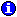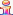﻿ In-line Math and Date Math

# In-line Math and Date Math

As the previous sections indicate, Pathagoras has the ability to perform simple mathematical calculations.

Your formulas can be stored in Input Form Masks (as previously shown). However, you can also insert math formulas 'in-line' with the source text The requisites are as follows:

The maximum number of operands is 4 (and by extension, the maximum number of actual calculations is 3)

Only addition, subtraction, multiplication and division calculations are possible.

If a formula contains variables, the precedent values of those variables must appear 'above' the formula.

If you are performing mixed date and 'standard' math calculations, the date calculations must come first. (see below for an example of a 'mixed' calculation)'. Processing proceeds from left to right

A precise structure is required (examples shown below), but it's really not that hard:

The 'formula' is:

1. Square brackets surrounding the entire variable term;

2. The call-to-action "#Math#" (no quotes) placed immediately after the opening bracket;

3. The variable name;

4. An equal sign to indicate the equivalency;

5. The formula, which is simply a reference to the existing variable, and the math you wish to perform. Note that there will always be a nesting of the variables, and the closing of the variable will very frequently be a double ']]'.

Here are some examples. The first elements are simply plain variables. They need to be completed in order for any in-line math to be performed. There is no order requirement EXCEPT that all references must be on the same Instant Database page as the formula.

 NOTE: The 'in-line' formulas discussed on this page are what appear 'in-line' in your text. They are just formulas that you would type into a Mask. When you invoke 'Scan', Pathagoras identifies the in-line formula and parses out the 'variable name' from the formula and places the components into the proper column on the Instant Database screen. The resulting entries are the same as if you manually typed a variable and a formula per the guidance provided in the preceding section.

Simple Math ('total price', 'half unit price' and 'half total price' are the target variables):

[Quantity]

[Price]

[#Math#total price=[Quantity]x[Price]]

[#Math#half unit price=[Price]/2]

[#Math#half total price=[Quantity]x[Price]/2]

(Note: multiplication may be indicated by 'x',or '*'. However a capital 'X" will not be seen as an operand. )

Date Math:

This contract will begin on [start date].

It will automatically renew on [#Math#termination date=[start date] + [3 months]], and every 3 months thereafter.

If you want to return the 'expiry' date (the day before the 'typical' return date, use this setup:

This contract will begin on [start date].

It will expire at midnight on [#Math#termination date=[start date] + [3 months] - [1 day]].

Here is an example of a 'mixed' calculation, first calculating a 'difference' in days between two dates, and then multiplying that difference by a penalty per day figure.

We requested the supporting documents on [Date Docs Requested] but to date have not received them.

Federal regulations require production of the documents within 30 days, and allow a penalty for each day the documents are not produced after that. Therefore, the penalty for the delay is <\$>[#Math#Penalty=[Today] - [Date Docs Requested] - [30 days] * 110] as of today's date.

A calculated date in the future, expressed in the 'extended'  the Xth date of Month, Year' format:

The notice was published on [Date of Notice].

If you do not respond by [#Math#Date Sale(ext)=[Date of Notice]+[21 days]], we shall proceed with the foreclosure sale

Age Math ('Age of H' is the target variable):

Husband was born on [Birthday of H].

He is [#Math#Age of H in years=[Today]-[Birthday of H]] years old.

Susie was born on [Susie Birthday]

Susie is [#Math#Age of Susie in years and months=[Today]-[Susie birthday]] old.

(Note: When the variable [Today] is in the formula, you need not pre-set its value. Pathagoras will assign the current date to the variable.One might say that the above formulas are pretty complex to set up. While that may be true, keep this in mind: Math in any program requires setting up a formula. With Pathagoras (as opposed to other programs), the formula is shown 'in-line' and on the face of the document. That makes it more accessible for editing, and more accessible for 'seeing' what is happening.Once the variable in the in-line formula has been 'calculated,' the variable can be used in other parts of the document without the 'formula'. So once 'total price' or 'half unit price' or 'termination date', etc. have been used and processed in a document, that variable can be referenced later in the document as a 'normal' variable, e.g.,  [total price] or [half unit price] or [termination date]. After all, it's just a plain text variable!By default, Pathagoras will return the calculated value in number of days. To return weeks, months or years, be sure to include the term 'weeks' 'months' or 'years' somewhere in the formula. E.g.,

John Smith worked for Spacely Sprockets for [#Math#duration in weeks=[Client 1 Empl Start Date] -[Client 1 Empl End Date]] weeks.